Colligative Properties

Learning Objectives

By the end of this section, you will be able to:

• Express concentrations of solution components using mole fraction and molality
• Describe the effect of solute concentration on various solution properties (vapor pressure, boiling point, freezing point, and osmotic pressure)
• Perform calculations using the mathematical equations that describe these various colligative effects
• Describe the process of distillation and its practical applications
• Explain the process of osmosis and describe how it is applied industrially and in nature

The properties of a solution are different from those of either the pure solute(s) or solvent. Many solution properties are dependent upon the chemical identity of the solute. Compared to pure water, a solution of hydrogen chloride is more acidic, a solution of ammonia is more basic, a solution of sodium chloride is more dense, and a solution of sucrose is more viscous. There are a few solution properties, however, that depend only upon the total concentration of solute species, regardless of their identities. These colligative properties include vapor pressure lowering, boiling point elevation, freezing point depression, and osmotic pressure. This small set of properties is of central importance to many natural phenomena and technological applications, as will be described in this module.

Mole Fraction and Molality

Several units commonly used to express the concentrations of solution components were introduced in an earlier chapter of this text, each providing certain benefits for use in different applications. For example, molarity (M) is a convenient unit for use in stoichiometric calculations, since it is defined in terms of the molar amounts of solute species:

$M=\frac{\text{mol solute}}{\text{L solution}}$

Because solution volumes vary with temperature, molar concentrations will likewise vary. When expressed as molarity, the concentration of a solution with identical numbers of solute and solvent species will be different at different temperatures, due to the contraction/expansion of the solution. More appropriate for calculations involving many colligative properties are mole-based concentration units whose values are not dependent on temperature. Two such units are mole fraction (introduced in the previous chapter on gases) and molality.

The mole fraction, X, of a component is the ratio of its molar amount to the total number of moles of all solution components:

${X}_{\text{A}}=\frac{\text{mol}\text{A}}{\text{total mol of all components}}$

Molality is a concentration unit defined as the ratio of the numbers of moles of solute to the mass of the solvent in kilograms:

$m=\frac{\text{mol solute}}{\text{kg solvent}}$

Since these units are computed using only masses and molar amounts, they do not vary with temperature and, thus, are better suited for applications requiring temperature-independent concentrations, including several colligative properties, as will be described in this chapter module.

Example 1: Calculating Mole Fraction and Molality

The antifreeze in most automobile radiators is a mixture of equal volumes of ethylene glycol and water, with minor amounts of other additives that prevent corrosion. What are the (a) mole fraction and (b) molality of ethylene glycol, C2H4(OH)2, in a solution prepared from 2.22 × 103 g of ethylene glycol and 2.00 × 103 g of water (approximately 2 L of glycol and 2 L of water)?

What are the mole fraction and molality of a solution that contains 0.850 g of ammonia, NH3, dissolved in 125 g of water?

Example 2: Converting Mole Fraction and Molal Concentrations

Calculate the mole fraction of solute and solvent in a 3.0 m solution of sodium chloride.

The mole fraction of iodine, I2, dissolved in dichloromethane, CH2Cl2, is 0.115. What is the molal concentration, m, of iodine in this solution?

Exercises

1. What are the mole fractions of H3PO4 and water in a solution of 14.5 g of H3PO4 in 125 g of water?
1. Outline the steps necessary to answer the question.
2. What are the mole fractions of HNO3 and water in a concentrated solution of nitric acid (68.0% HNO3 by mass)?
1. Outline the steps necessary to answer the question.
3. Calculate the mole fraction of each solute and solvent:
1. 583 g of H2SO4 in 1.50 kg of water—the acid solution used in an automobile battery
2. 0.86 g of NaCl in 1.00 × 102 g of water—a solution of sodium chloride for intravenous injection
3. 46.85 g of codeine, C18H21NO3, in 125.5 g of ethanol, C2H5OH
4. 25 g of I2 in 125 g of ethanol, C2H5OH
4. Calculate the mole fraction of each solute and solvent:
1. 0.710 kg of sodium carbonate (washing soda), Na2CO3, in 10.0 kg of water—a saturated solution at 0 °C
2. 125 g of NH4NO3 in 275 g of water—a mixture used to make an instant ice pack
3. 25 g of Cl2 in 125 g of dichloromethane, CH2Cl
4. 0.372 g of histamine, C5H9N, in 125 g of chloroform, CHCl3
5. Calculate the mole fractions of methanol, CH3OH; ethanol, C2H5OH; and water in a solution that is 40% methanol, 40% ethanol, and 20% water by mass. (Assume the data are good to two significant figures.)
6. What is the difference between a 1 M solution and a 1 m solution?
7. What is the molality of phosphoric acid, H3PO4, in a solution of 14.5 g of H3PO4 in 125 g of water?
1. Outline the steps necessary to answer the question.
8. What is the molality of nitric acid in a concentrated solution of nitric acid (68.0% HNO3 by mass)?
1. Outline the steps necessary to answer the question.
9. Calculate the molality of each of the following solutions:
1. 583 g of H2SO4 in 1.50 kg of water—the acid solution used in an automobile battery
2. 0.86 g of NaCl in 1.00 × 102 g of water—a solution of sodium chloride for intravenous injection
3. 46.85 g of codeine, C18H21NO3, in 125.5 g of ethanol, C2H5OH
4. 25 g of I2 in 125 g of ethanol, C2H5OH
10. Calculate the molality of each of the following solutions:
1. 0.710 kg of sodium carbonate (washing soda), Na2CO3, in 10.0 kg of water—a saturated solution at 0°C
2. 125 g of NH4NO3 in 275 g of water—a mixture used to make an instant ice pack
3. 25 g of Cl2 in 125 g of dichloromethane, CH2Cl
4. 0.372 g of histamine, C5H9N, in 125 g of chloroform, CHCl3
11. The concentration of glucose, C6H12O6, in normal spinal fluid is $\frac{75\text{mg}}{100\text{g}}$. What is the molality of the solution?
12. A 13.0% solution of K2CO3 by mass has a density of 1.09 g/cm3. Calculate the molality of the solution.

Vapor Pressure Lowering

As described in the chapter on liquids and solids, the equilibrium vapor pressure of a liquid is the pressure exerted by its gaseous phase when vaporization and condensation are occurring at equal rates:

$\text{liquid}\rightleftharpoons\text{gas}$

Dissolving a nonvolatile substance in a volatile liquid results in a lowering of the liquid’s vapor pressure. This phenomenon can be rationalized by considering the effect of added solute molecules on the liquid’s vaporization and condensation processes. To vaporize, solvent molecules must be present at the surface of the solution. The presence of solute decreases the surface area available to solvent molecules and thereby reduces the rate of solvent vaporization. Since the rate of condensation is unaffected by the presence of solute, the net result is that the vaporization-condensation equilibrium is achieved with fewer solvent molecules in the vapor phase (i.e., at a lower vapor pressure) (Figure 1). While this kinetic interpretation is useful, it does not account for several important aspects of the colligative nature of vapor pressure lowering. A more rigorous explanation involves the property of entropy, a topic of discussion in a later text chapter on thermodynamics. For purposes of understanding the lowering of a liquid’s vapor pressure, it is adequate to note that the greater entropy of a solution in comparison to its separate solvent and solute serves to effectively stabilize the solvent molecules and hinder their vaporization. A lower vapor pressure results, and a correspondingly higher boiling point as described in the next section of this module.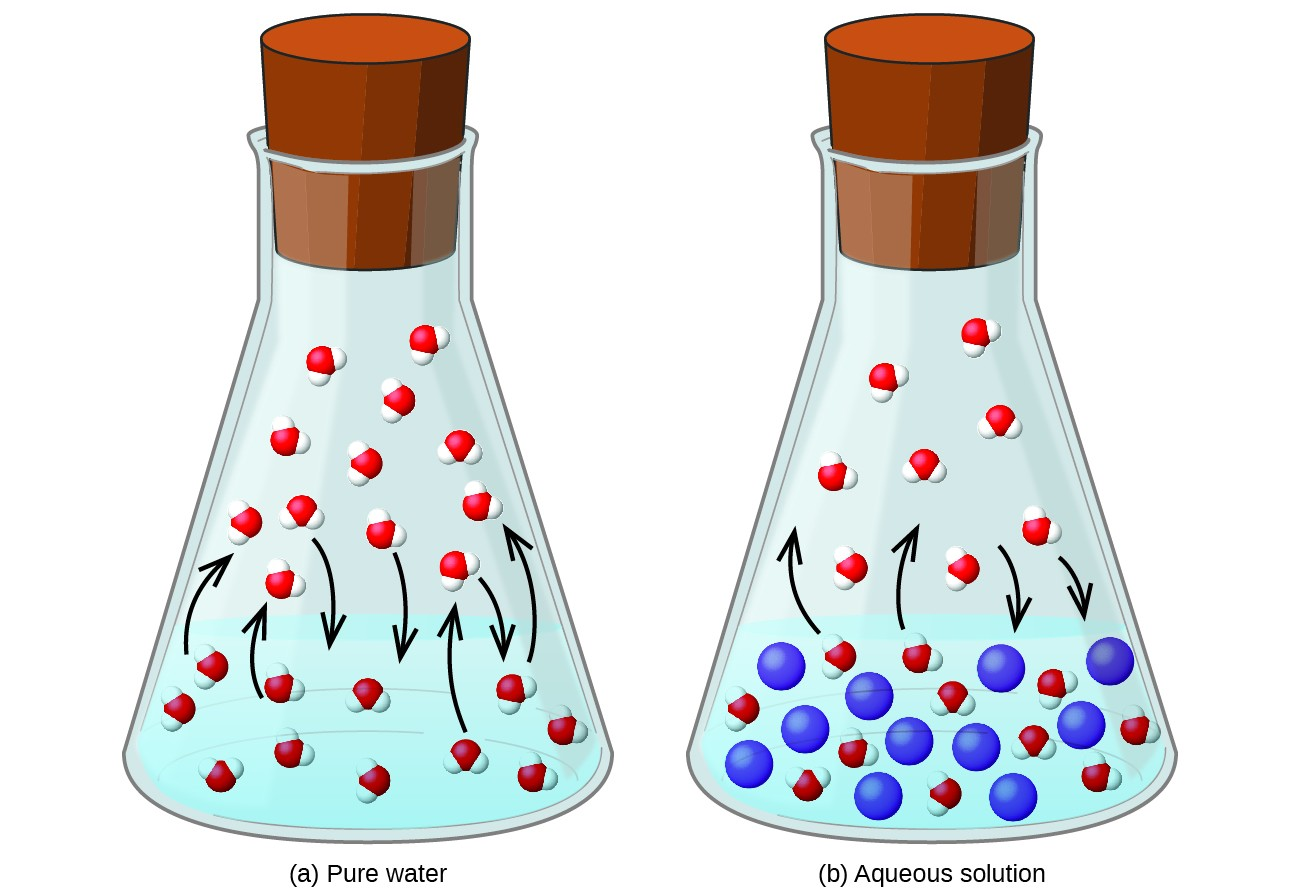Figure 1. The presence of nonvolatile solutes lowers the vapor pressure of a solution by impeding the evaporation of solvent molecules.

The relationship between the vapor pressures of solution components and the concentrations of those components is described by Raoult’s law: The partial pressure exerted by any component of an ideal solution is equal to the vapor pressure of the pure component multiplied by its mole fraction in the solution.

${P}_{\text{A}}={X}_{\text{A}}{P}_{\text{A}}^{ \circ }$

where PA is the partial pressure exerted by component A in the solution, ${P}_{\text{A}}^{\circ }$ is the vapor pressure of pure A, and XA is the mole fraction of A in the solution. (Mole fraction is a concentration unit introduced in the chapter on gases.)

Recalling that the total pressure of a gaseous mixture is equal to the sum of partial pressures for all its components (Dalton’s law of partial pressures), the total vapor pressure exerted by a solution containing i components is

${P}_{\text{solution}}=\sum _{i}{P}_{i}=\sum _{i}{X}_{i}{P}_{i}^{\circ}$

A nonvolatile substance is one whose vapor pressure is negligible (P° ≈ 0), and so the vapor pressure above a solution containing only nonvolatile solutes is due only to the solvent:

${P}_{\text{solution}}={X}_{\text{solvent}}{P}_{\text{solvent}}^{\circ }$

Example 3: Calculation of a Vapor Pressure

Compute the vapor pressure of an ideal solution containing 92.1 g of glycerin, C3H5(OH)3, and 184.4 g of ethanol, C2H5OH, at 40 °C. The vapor pressure of pure ethanol is 0.178 atm at 40 °C. Glycerin is essentially nonvolatile at this temperature.

A solution contains 5.00 g of urea, CO(NH2)2 (a nonvolatile solute) and 0.100 kg of water. If the vapor pressure of pure water at 25 °C is 23.7 torr, what is the vapor pressure of the solution?

Elevation of the Boiling Point of a Solvent

As described in the chapter on liquids and solids, the boiling point of a liquid is the temperature at which its vapor pressure is equal to ambient atmospheric pressure. Since the vapor pressure of a solution is lowered due to the presence of nonvolatile solutes, it stands to reason that the solution’s boiling point will subsequently be increased. Compared to pure solvent, a solution, therefore, will require a higher temperature to achieve any given vapor pressure, including one equivalent to that of the surrounding atmosphere. The increase in boiling point observed when nonvolatile solute is dissolved in a solvent, ΔTb, is called boiling point elevation and is directly proportional to the molal concentration of solute species:

$\Delta{T}_{\text{b}}={K}_{\text{b}}m$

where Kb is the boiling point elevation constant, or the ebullioscopic constant and m is the molal concentration (molality) of all solute species.

Boiling point elevation constants are characteristic properties that depend on the identity of the solvent. Values of Kb for several solvents are listed in Table 1.

Table 1. Boiling Point Elevation and Freezing Point Depression Constants for Several Solvents
Solvent Boiling Point (°C at 1 atm) Kb (Cm−1) Freezing Point (°C at 1 atm) Kf (Cm−1)
water 100.0 0.512 0.0 1.86
hydrogen acetate 118.1 3.07 16.6 3.9
benzene 80.1 2.53 5.5 5.12
chloroform 61.26 3.63 −63.5 4.68
nitrobenzene 210.9 5.24 5.67 8.1

The extent to which the vapor pressure of a solvent is lowered and the boiling point is elevated depends on the total number of solute particles present in a given amount of solvent, not on the mass or size or chemical identities of the particles. A 1 m aqueous solution of sucrose (342 g/mol) and a 1 m aqueous solution of ethylene glycol (62 g/mol) will exhibit the same boiling point because each solution has one mole of solute particles (molecules) per kilogram of solvent.

Example 4: Calculating the Boiling Point of a Solution

What is the boiling point of a 0.33 m solution of a nonvolatile solute in benzene?

What is the boiling point of the antifreeze described in Example 1?

Example 5: The Boiling Point of an Iodine Solution

Find the boiling point of a solution of 92.1 g of iodine, I2, in 800.0 g of chloroform, CHCl3, assuming that the iodine is nonvolatile and that the solution is ideal.

What is the boiling point of a solution of 1.0 g of glycerin, C3H5(OH)3, in 47.8 g of water? Assume an ideal solution.

Exercises

1. Why does 1 mol of sodium chloride depress the freezing point of 1 kg of water almost twice as much as 1 mol of glycerin?
2. What is the boiling point of a solution of 115.0 g of sucrose, C12H22O11, in 350.0 g of water?
1. Outline the steps necessary to answer the question
3. What is the boiling point of a solution of 9.04 g of I2 in 75.5 g of benzene, assuming the I2 is nonvolatile?
1. Outline the steps necessary to answer the question.
4. What is the freezing temperature of a solution of 115.0 g of sucrose, C12H22O11, in 350.0 g of water, which freezes at 0.0 °C when pure?
1. Outline the steps necessary to answer the question.
5. What is the freezing point of a solution of 9.04 g of I2 in 75.5 g of benzene?
1. Outline the steps necessary to answer the following question.

Distillation of Solutions

Distillation is a technique for separating the components of mixtures that is widely applied in both in the laboratory and in industrial settings. It is used to refine petroleum, to isolate fermentation products, and to purify water. This separation technique involves the controlled heating of a sample mixture to selectively vaporize, condense, and collect one or more components of interest. A typical apparatus for laboratory-scale distillations is shown in Figure 2.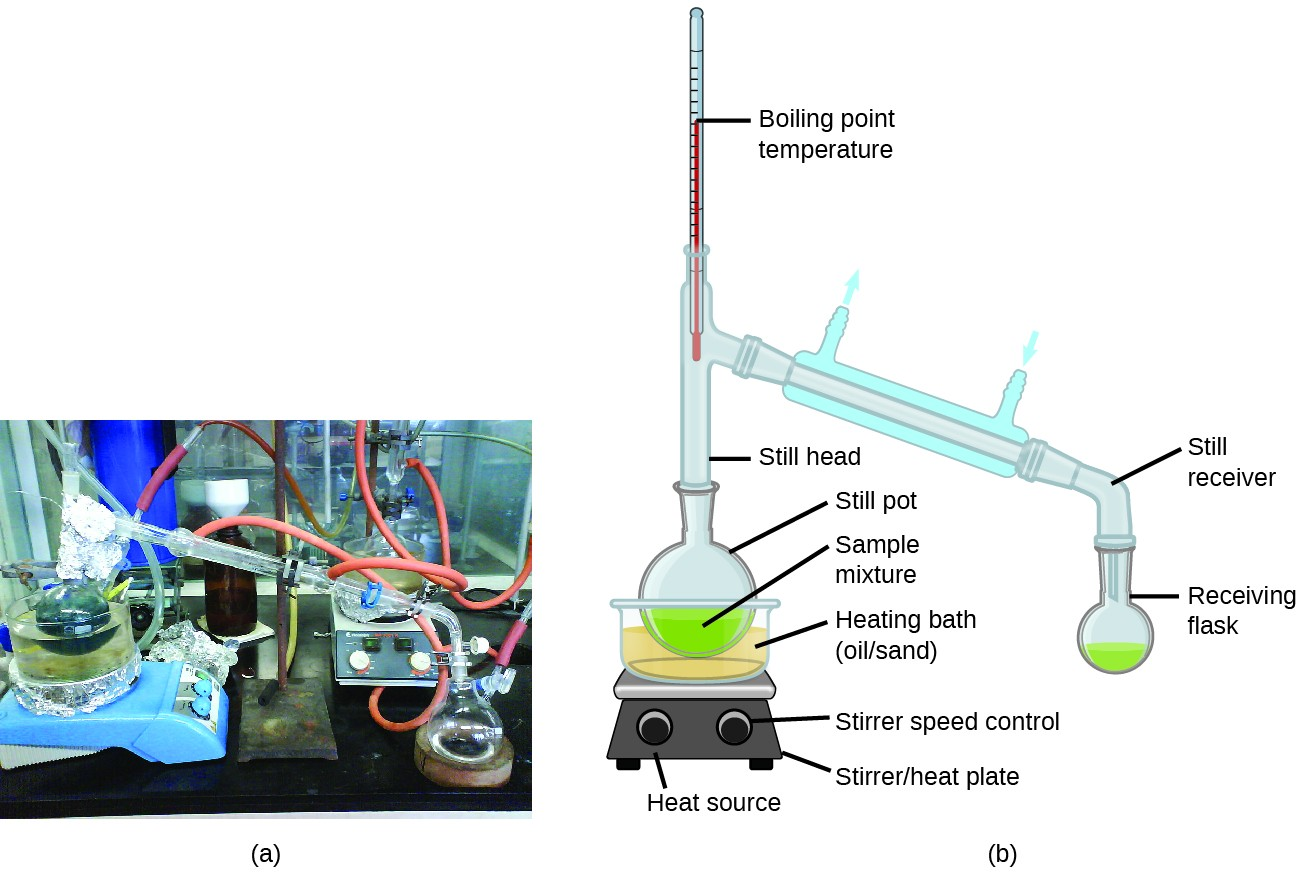Figure 2. A typical laboratory distillation unit is shown in (a) a photograph and (b) a schematic diagram of the components. (credit a: modification of work by “Rifleman82”/Wikimedia commons; credit b: modification of work by “Slashme”/Wikipedia)

Oil refineries use large-scale fractional distillation to separate the components of crude oil. The crude oil is heated to high temperatures at the base of a tall fractionating column, vaporizing many of the components that rise within the column. As vaporized components reach adequately cool zones during their ascent, they condense and are collected. The collected liquids are simpler mixtures of hydrocarbons and other petroleum compounds that are of appropriate composition for various applications (e.g., diesel fuel, kerosene, gasoline), as depicted in Figure 3.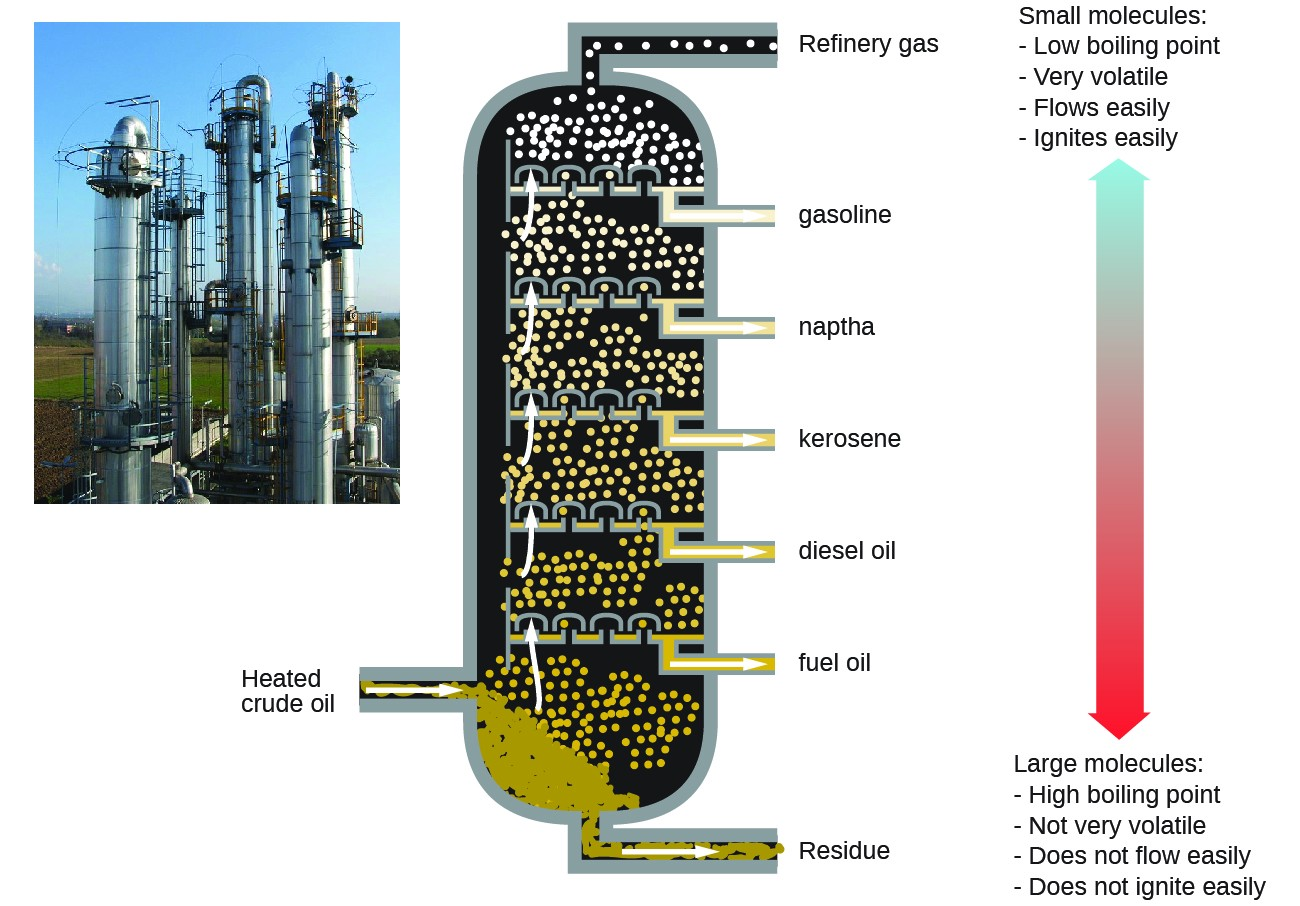Figure 3. Crude oil is a complex mixture that is separated by large-scale fractional distillation to isolate various simpler mixtures.

Depression of the Freezing Point of a Solvent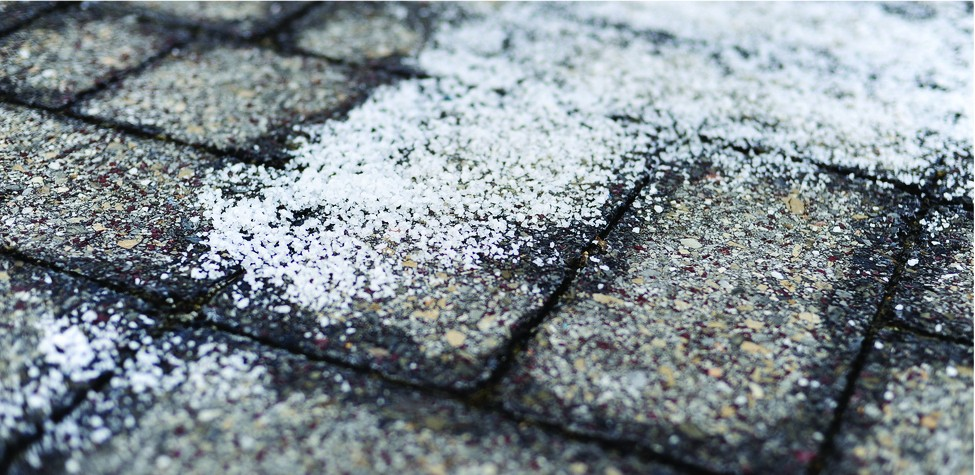Figure 4. Rock salt (NaCl), calcium chloride (CaCl2), or a mixture of the two are used to melt ice. (credit: modification of work by Eddie Welker)

Solutions freeze at lower temperatures than pure liquids. This phenomenon is exploited in “de-icing” schemes that use salt (Figure 4), calcium chloride, or urea to melt ice on roads and sidewalks, and in the use of ethylene glycol as an “antifreeze” in automobile radiators. Seawater freezes at a lower temperature than fresh water, and so the Arctic and Antarctic oceans remain unfrozen even at temperatures below 0 °C (as do the body fluids of fish and other cold-blooded sea animals that live in these oceans).

The decrease in freezing point of a dilute solution compared to that of the pure solvent, ΔTf, is called the freezing point depression and is directly proportional to the molal concentration of the solute

$\Delta{T}_{\text{f}}={K}_{\text{f}}m$

where m is the molal concentration of the solute in the solvent and Kf is called the freezing point depression constant (or cryoscopic constant). Just as for boiling point elevation constants, these are characteristic properties whose values depend on the chemical identity of the solvent. Values of Kf for several solvents are listed in Table 1.

Example 6: Calculation of the Freezing Point of a Solution

What is the freezing point of the 0.33 m solution of a nonvolatile nonelectrolyte solute in benzene described in Example 2?

What is the freezing point of a 1.85 m solution of a nonvolatile nonelectrolyte solute in nitrobenzene?

Colligative Properties and De-Icing

Sodium chloride and its group 2 analogs calcium and magnesium chloride are often used to de-ice roadways and sidewalks, due to the fact that a solution of any one of these salts will have a freezing point lower than 0 °C, the freezing point of pure water. The group 2 metal salts are frequently mixed with the cheaper and more readily available sodium chloride (“rock salt”) for use on roads, since they tend to be somewhat less corrosive than the NaCl, and they provide a larger depression of the freezing point, since they dissociate to yield three particles per formula unit, rather than two particles like the sodium chloride.

Because these ionic compounds tend to hasten the corrosion of metal, they would not be a wise choice to use in antifreeze for the radiator in your car or to de-ice a plane prior to takeoff. For these applications, covalent compounds, such as ethylene or propylene glycol, are often used. The glycols used in radiator fluid not only lower the freezing point of the liquid, but they elevate the boiling point, making the fluid useful in both winter and summer. Heated glycols are often sprayed onto the surface of airplanes prior to takeoff in inclement weather in the winter to remove ice that has already formed and prevent the formation of more ice, which would be particularly dangerous if formed on the control surfaces of the aircraft (Figure 5).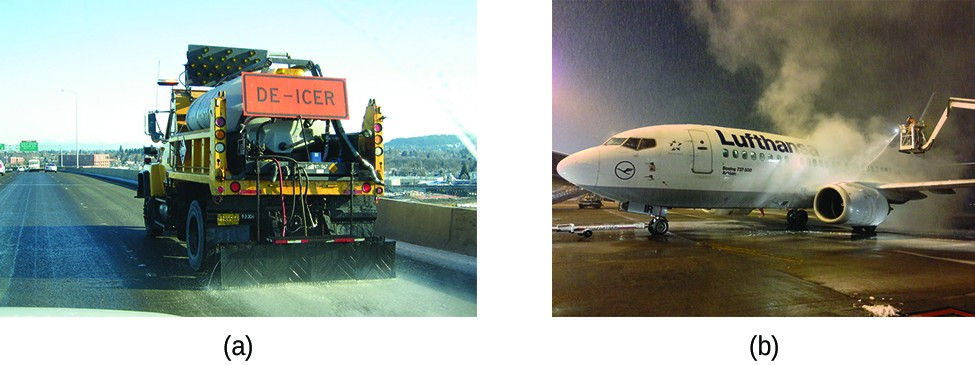Figure 5. Freezing point depression is exploited to remove ice from (a) roadways and (b) the control surfaces of aircraft.

Exercises

1. A 1.0 m solution of HCl in benzene has a freezing point of 0.4 °C. Is HCl an electrolyte in benzene? Explain.
2. A 12.0-g sample of a nonelectrolyte is dissolved in 80.0 g of water. The solution freezes at -1.94 °C. Calculate the molar mass of the substance.
3. Calculate the boiling point elevation of 0.100 kg of water containing 0.010 mol of NaCl, 0.020 mol of Na2SO4, and 0.030 mol of MgCl2, assuming complete dissociation of these electrolytes.

Phase Diagram for an Aqueous Solution of a Nonelectrolyte

The colligative effects on vapor pressure, boiling point, and freezing point described in the previous section are conveniently summarized by comparing the phase diagrams for a pure liquid and a solution derived from that liquid. Phase diagrams for water and an aqueous solution are shown in Figure 6.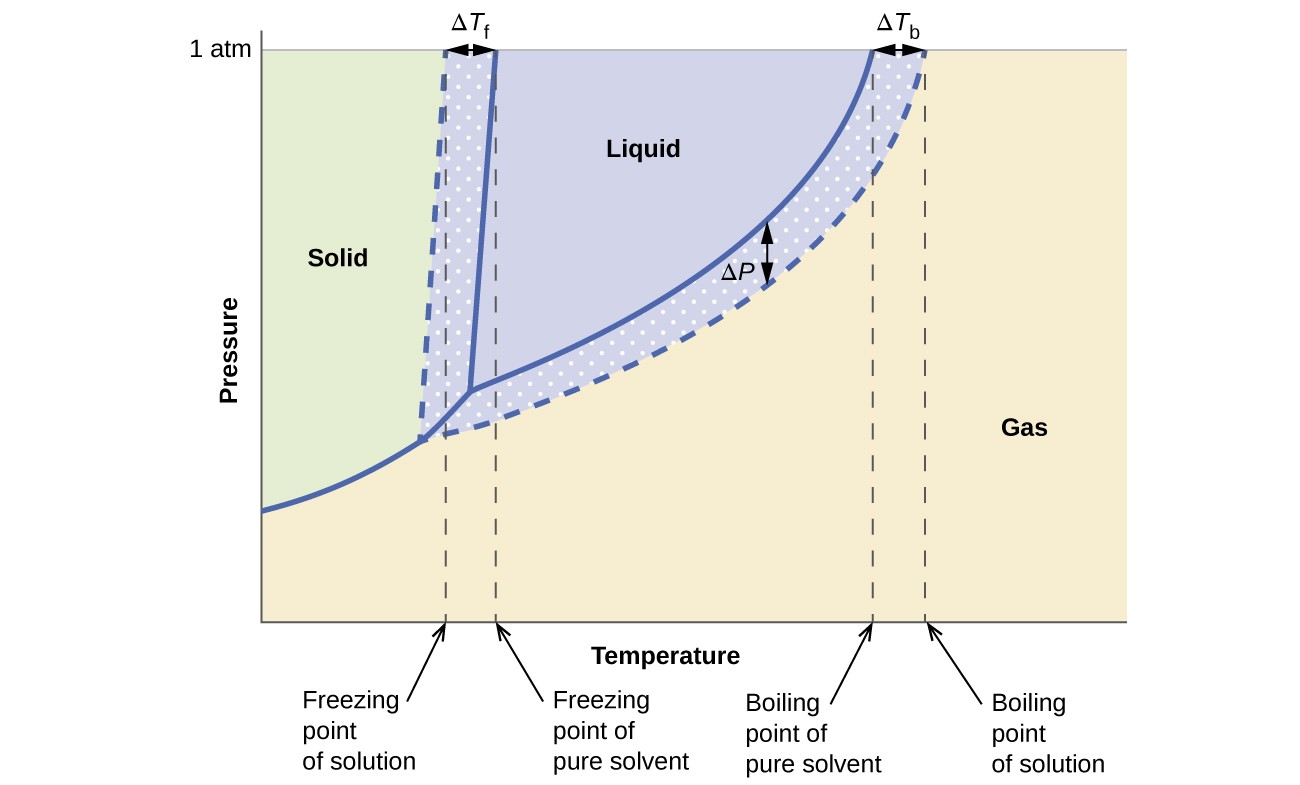Figure 6. These phase diagrams show water (solid curves) and an aqueous solution of nonelectrolyte (dashed curves).

The liquid-vapor curve for the solution is located beneath the corresponding curve for the solvent, depicting the vapor pressure lowering, ΔP, that results from the dissolution of nonvolatile solute. Consequently, at any given pressure, the solution’s boiling point is observed at a higher temperature than that for the pure solvent, reflecting the boiling point elevation, ΔTb, associated with the presence of nonvolatile solute. The solid-liquid curve for the solution is displaced left of that for the pure solvent, representing the freezing point depression, ΔTb, that accompanies solution formation. Finally, notice that the solid-gas curves for the solvent and its solution are identical. This is the case for many solutions comprising liquid solvents and nonvolatile solutes. Just as for vaporization, when a solution of this sort is frozen, it is actually just the solvent molecules that undergo the liquid-to-solid transition, forming pure solid solvent that excludes solute species. The solid and gaseous phases, therefore, are composed solvent only, and so transitions between these phases are not subject to colligative effects.

Osmosis and Osmotic Pressure of Solutions

A number of natural and synthetic materials exhibit selective permeation, meaning that only molecules or ions of a certain size, shape, polarity, charge, and so forth, are capable of passing through (permeating) the material. Biological cell membranes provide elegant examples of selective permeation in nature, while dialysis tubing used to remove metabolic wastes from blood is a more simplistic technological example. Regardless of how they may be fabricated, these materials are generally referred to as semipermeable membranes.

Consider the apparatus illustrated in Figure 7, in which samples of pure solvent and a solution are separated by a membrane that only solvent molecules may permeate. Solvent molecules will diffuse across the membrane in both directions. Since the concentration of solvent is greater in the pure solvent than the solution, these molecules will diffuse from the solvent side of the membrane to the solution side at a faster rate than they will in the reverse direction. The result is a net transfer of solvent molecules from the pure solvent to the solution. Diffusion-driven transfer of solvent molecules through a semipermeable membrane is a process known as osmosis.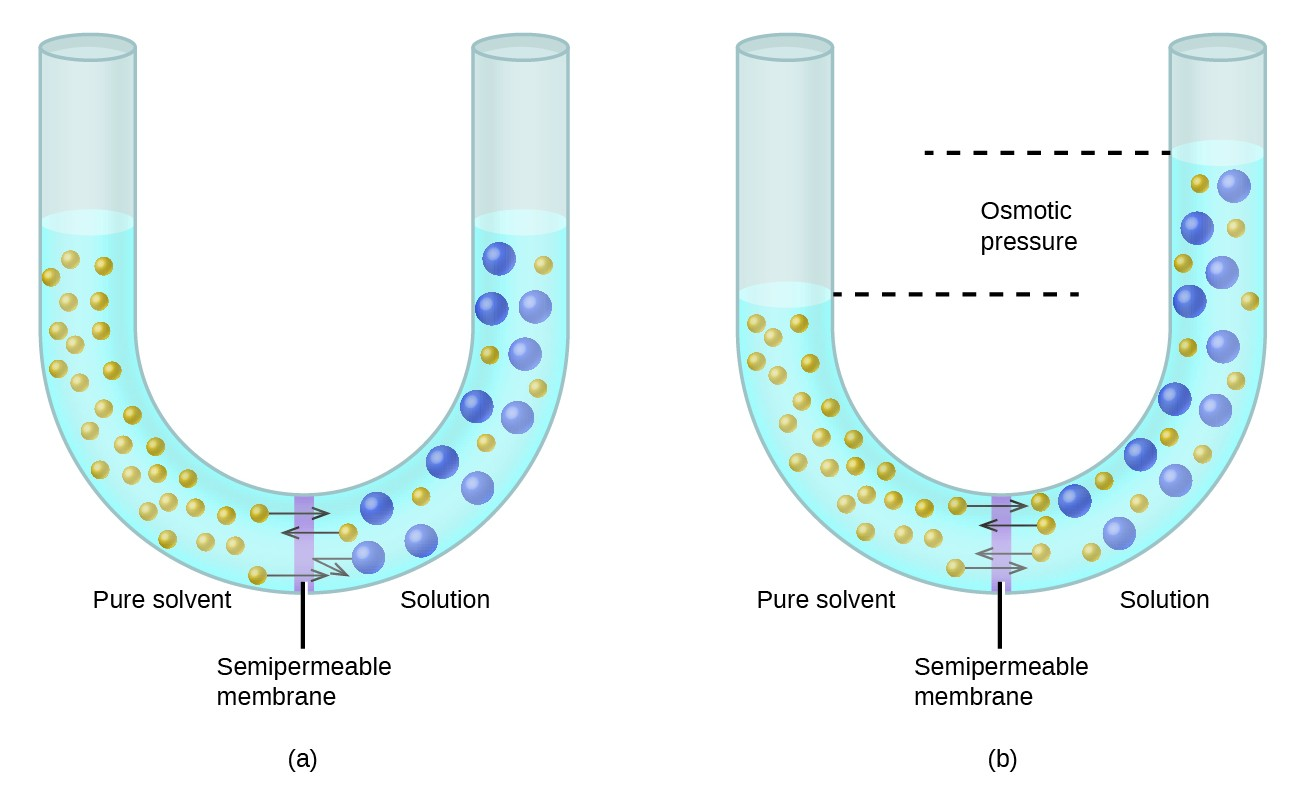Figure 7. Osmosis results in the transfer of solvent molecules from a sample of low (or zero) solute concentration to a sample of higher solute concentration.

When osmosis is carried out in an apparatus like that shown in Figure 7, the volume of the solution increases as it becomes diluted by accumulation of solvent. This causes the level of the solution to rise, increasing its hydrostatic pressure (due to the weight of the column of solution in the tube) and resulting in a faster transfer of solvent molecules back to the pure solvent side. When the pressure reaches a value that yields a reverse solvent transfer rate equal to the osmosis rate, bulk transfer of solvent ceases. This pressure is called the osmotic pressure (Π) of the solution. The osmotic pressure of a dilute solution is related to its solute molarity, M, and absolute temperature, T, according to the equation

$\Pi =MRT$

where R is the universal gas constant.

Example 7: Calculation of Osmotic Pressure

What is the osmotic pressure (atm) of a 0.30 M solution of glucose in water that is used for intravenous infusion at body temperature, 37 °C?

What is the osmotic pressure (atm) a solution with a volume of 0.750 L that contains 5.0 g of methanol, CH3OH, in water at 37 °C?

If a solution is placed in an apparatus like the one shown in Figure 8, applying pressure greater than the osmotic pressure of the solution reverses the osmosis and pushes solvent molecules from the solution into the pure solvent. This technique of reverse osmosis is used for large-scale desalination of seawater and on smaller scales to produce high-purity tap water for drinking.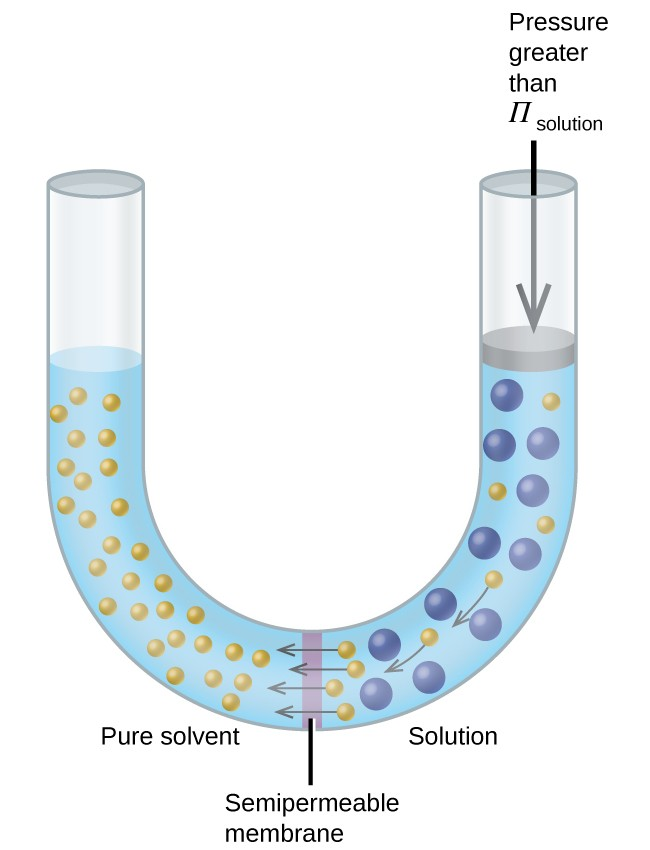Figure 8. Applying a pressure greater than the osmotic pressure of a solution will reverse osmosis. Solvent molecules from the solution are pushed into the pure solvent.

Reverse Osmosis Water Purification

In the process of osmosis, diffusion serves to move water through a semipermeable membrane from a less concentrated solution to a more concentrated solution. Osmotic pressure is the amount of pressure that must be applied to the more concentrated solution to cause osmosis to stop. If greater pressure is applied, the water will go from the more concentrated solution to a less concentrated (more pure) solution. This is called reverse osmosis. Reverse osmosis (RO) is used to purify water in many applications, from desalination plants in coastal cities, to water-purifying machines in grocery stores (Figure 9), and smaller reverse-osmosis household units. With a hand-operated pump, small RO units can be used in third-world countries, disaster areas, and in lifeboats. Our military forces have a variety of generator-operated RO units that can be transported in vehicles to remote locations.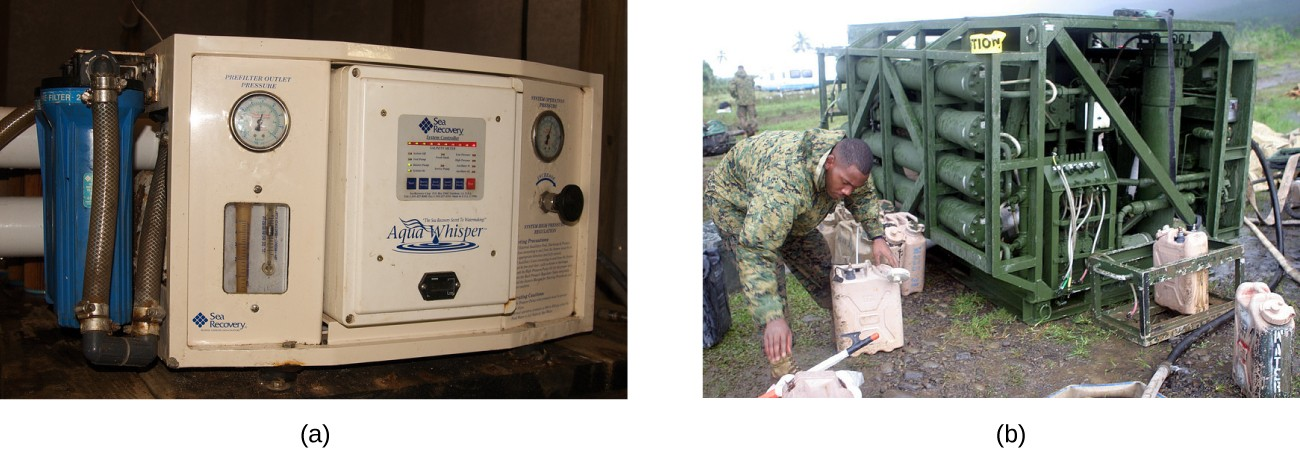Figure 9. Reverse osmosis systems for purifying drinking water are shown here on (a) small and (b) large scales. (credit a: modification of work by Jerry Kirkhart; credit b: modification of work by Willard J. Lathrop)

Examples of osmosis are evident in many biological systems because cells are surrounded by semipermeable membranes. Carrots and celery that have become limp because they have lost water can be made crisp again by placing them in water. Water moves into the carrot or celery cells by osmosis. A cucumber placed in a concentrated salt solution loses water by osmosis and absorbs some salt to become a pickle. Osmosis can also affect animal cells. Solute concentrations are particularly important when solutions are injected into the body. Solutes in body cell fluids and blood serum give these solutions an osmotic pressure of approximately 7.7 atm. Solutions injected into the body must have the same osmotic pressure as blood serum; that is, they should be isotonic with blood serum. If a less concentrated solution, a hypotonic solution, is injected in sufficient quantity to dilute the blood serum, water from the diluted serum passes into the blood cells by osmosis, causing the cells to expand and rupture. This process is called hemolysis. When a more concentrated solution, a hypertonic solution, is injected, the cells lose water to the more concentrated solution, shrivel, and possibly die in a process called crenation. These effects are illustrated in Figure 10.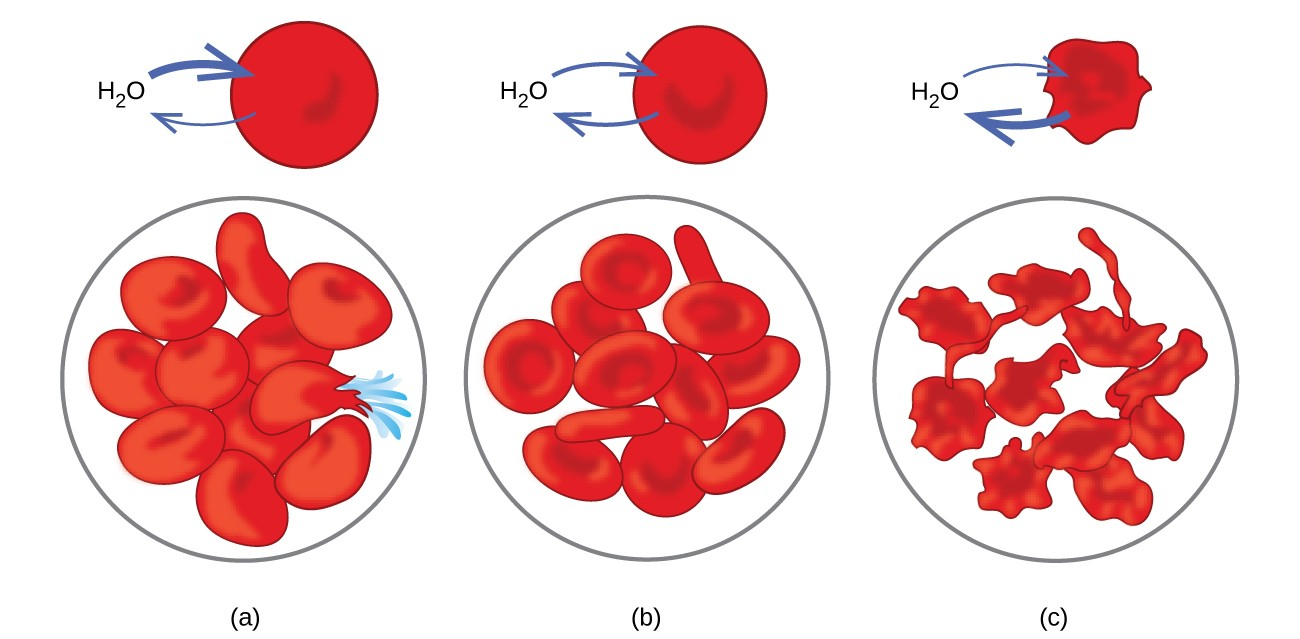Figure 10. Red blood cell membranes are water permeable and will (a) swell and possibly rupture in a hypotonic solution; (b) maintain normal volume and shape in an isotonic solution; and (c) shrivel and possibly die in a hypertonic solution. (credit a/b/c: modifications of work by “LadyofHats”/Wikimedia commons)

Exercises

1. What is the osmotic pressure of an aqueous solution of 1.64 g of Ca(NO3)2 in water at 25 °C? The volume of the solution is 275 mL.
1. Outline the steps necessary to answer the question.
2. What is osmotic pressure of a solution of bovine insulin (molar mass, 5700 g mol−1) at 18 °C if 100.0 mL of the solution contains 0.103 g of the insulin?
1. Outline the steps necessary to answer the question.

Determination of Molar Masses

Osmotic pressure and changes in freezing point, boiling point, and vapor pressure are directly proportional to the concentration of solute present. Consequently, we can use a measurement of one of these properties to determine the molar mass of the solute from the measurements.

Example 8: Determination of a Molar Mass from a Freezing Point Depression

A solution of 4.00 g of a nonelectrolyte dissolved in 55.0 g of benzene is found to freeze at 2.32 °C. What is the molar mass of this compound?

A solution of 35.7 g of a nonelectrolyte in 220.0 g of chloroform has a boiling point of 64.5 °C. What is the molar mass of this compound?

Example 9: Determination of a Molar Mass from Osmotic Pressure

A 0.500 L sample of an aqueous solution containing 10.0 g of hemoglobin has an osmotic pressure of 5.9 torr at 22 °C. What is the molar mass of hemoglobin?

What is the molar mass of a protein if a solution of 0.02 g of the protein in 25.0 mL of solution has an osmotic pressure of 0.56 torr at 25 °C?

Exercises

1. What is the molar mass of a solution of 5.00 g of a compound in 25.00 g of carbon tetrachloride (bp 76.8 °C; Kb = 5.02 °C/m) that boils at 81.5 °C at 1 atm?
1. Outline the steps necessary to answer the question.
2. Solve the problem.
2. A sample of an organic compound (a nonelectrolyte) weighing 1.35 g lowered the freezing point of 10.0 g of benzene by 3.66 °C. Calculate the molar mass of the compound.

Colligative Properties of Electrolytes

As noted previously in this module, the colligative properties of a solution depend only on the number, not on the kind, of solute species dissolved. For example, 1 mole of any nonelectrolyte dissolved in 1 kilogram of solvent produces the same lowering of the freezing point as does 1 mole of any other nonelectrolyte. However, 1 mole of sodium chloride (an electrolyte) forms 2 moles of ions when dissolved in solution. Each individual ion produces the same effect on the freezing point as a single molecule does.

Example 10: The Freezing Point of a Solution of an Electrolyte

The concentration of ions in seawater is approximately the same as that in a solution containing 4.2 g of NaCl dissolved in 125 g of water. Assume that each of the ions in the NaCl solution has the same effect on the freezing point of water as a nonelectrolyte molecule, and determine the freezing temperature the solution (which is approximately equal to the freezing temperature of seawater).

Assume that each of the ions in calcium chloride, CaCl2, has the same effect on the freezing point of water as a nonelectrolyte molecule. Calculate the freezing point of a solution of 0.724 g of CaCl2 in 175 g of water.

Assuming complete dissociation, a 1.0 m aqueous solution of NaCl contains 1.0 mole of ions (1.0 mol Na+ and 1.0 mol Cl) per each kilogram of water, and its freezing point depression is expected to be

$\Delta{T}_{\text{f}}=2.0\text{mol ions/kg water}\times 1.86^{\circ}\text{C kg water/mol ion}=3.7^{\circ}\text{C}$

When this solution is actually prepared and its freezing point depression measured, however, a value of 3.4 °C is obtained. Similar discrepancies are observed for other ionic compounds, and the differences between the measured and expected colligative property values typically become more significant as solute concentrations increase. These observations suggest that the ions of sodium chloride (and other strong electrolytes) are not completely dissociated in solution.

To account for this and avoid the errors accompanying the assumption of total dissociation, an experimentally measured parameter named in honor of Nobel Prize-winning German chemist Jacobus Henricus van’t Hoff is used. The van’t Hoff factor (i) is defined as the ratio of solute particles in solution to the number of formula units dissolved:

$i=\frac{\text{moles of particles in solution}}{\text{moles of formula units dissolved}}$

Values for measured van’t Hoff factors for several solutes, along with predicted values assuming complete dissociation, are shown in Table 2.

Table 2. Expected and Observed van’t Hoff Factors for Several 0.050 m Aqueous Electrolyte Solutions
Electrolyte Particles in Solution i (Predicted) i (Measured)
HCl H+, Cl 2 1.9
NaCl Na+, Cl 2 1.9
MgSO4 Mg2+, ${\text{SO}}_{4}{}^{2-}$ 2 1.3
MgCl2 Mg2+, 2Cl 3 2.7
FeCl3 Fe3+, 3Cl 4 3.4
glucose C12H22O11 1 1.0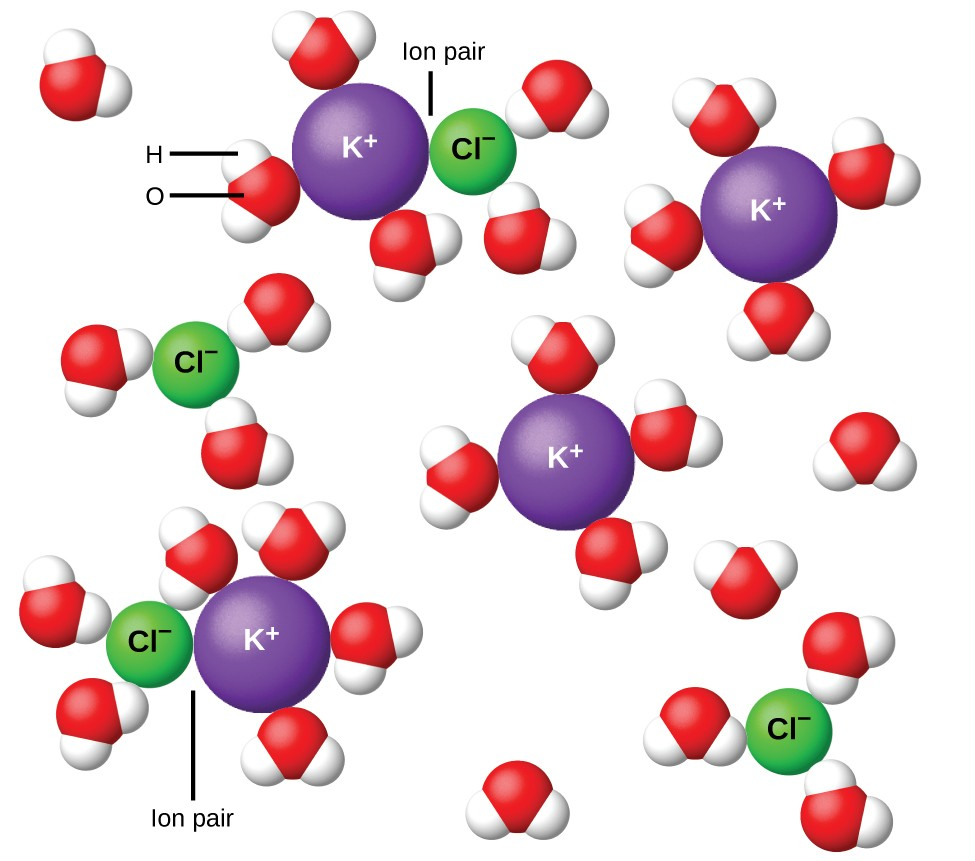Figure 11. Ions become more and more widely separated the more dilute the solution, and the residual interionic attractions become less.

In 1923, the chemists Peter Debye and Erich Hückel proposed a theory to explain the apparent incomplete ionization of strong electrolytes. They suggested that although interionic attraction in an aqueous solution is very greatly reduced by solvation of the ions and the insulating action of the polar solvent, it is not completely nullified. The residual attractions prevent the ions from behaving as totally independent particles (Figure 11). In some cases, a positive and negative ion may actually touch, giving a solvated unit called an ion pair. Thus, the activity, or the effective concentration, of any particular kind of ion is less than that indicated by the actual concentration. Ions become more and more widely separated the more dilute the solution, and the residual interionic attractions become less and less. Thus, in extremely dilute solutions, the effective concentrations of the ions (their activities) are essentially equal to the actual concentrations. Note that the van’t Hoff factors for the electrolytes in Table 2 are for 0.05 m solutions, at which concentration the value of i for NaCl is 1.9, as opposed to an ideal value of 2.

Exercises

1. Meat can be classified as fresh (not frozen) even though it is stored at −1 °C. Why wouldn’t meat freeze at this temperature?
2. An organic compound has a composition of 93.46% C and 6.54% H by mass. A solution of 0.090 g of this compound in 1.10 g of camphor melts at 158.4 °C. The melting point of pure camphor is 178.4 °C. Kf for camphor is 37.7 °C/m. What is the molecular formula of the solute? Show your calculations.
3. A salt is known to be an alkali metal fluoride. A quick approximate determination of freezing point indicates that 4 g of the salt dissolved in 100 g of water produces a solution that freezes at about −1.4 °C. What is the formula of the salt? Show your calculations.

Key Concepts and Summary

Properties of a solution that depend only on the concentration of solute particles are called colligative properties. They include changes in the vapor pressure, boiling point, and freezing point of the solvent in the solution. The magnitudes of these properties depend only on the total concentration of solute particles in solution, not on the type of particles. The total concentration of solute particles in a solution also determines its osmotic pressure. This is the pressure that must be applied to the solution to prevent diffusion of molecules of pure solvent through a semipermeable membrane into the solution. Ionic compounds may not completely dissociate in solution due to activity effects, in which case observed colligative effects may be less than predicted.

Key Equations

• $\left({P}_{\text{A}}={X}_{\text{A}}{P}_{\text{A}}^{\circ}\right)$
• ${P}_{\text{solution}}=\sum _{i}{P}_{i}=\sum _{i}{X}_{i}{P}_{i}^{\circ}$
• ${P}_{\text{solution}}={X}_{\text{solvent}}{P}_{\text{solvent}}^{\circ}$
• ΔTb = Kbm
• ΔTf = Kfm
• Π = MRT

Exercises

1. Which is/are part of the macroscopic domain of solutions and which is/are part of the microscopic domain: boiling point elevation, Henry’s law, hydrogen bond, ion-dipole attraction, molarity, nonelectrolyte, nonstoichiometric compound, osmosis, solvated ion?
2. What is the microscopic explanation for the macroscopic behavior illustrated in Figure 7 in Solubility?
3. Sketch a qualitative graph of the pressure versus time for water vapor above a sample of pure water and a sugar solution, as the liquids evaporate to half their original volume.
4. A solution of potassium nitrate, an electrolyte, and a solution of glycerin (C3H5(OH)3), a nonelectrolyte, both boil at 100.3 °C. What other physical properties of the two solutions are identical?
5. A solution contains 5.00 g of urea, CO(NH2)2, a nonvolatile compound, dissolved in 0.100 kg of water. If the vapor pressure of pure water at 25 °C is 23.7 torr, what is the vapor pressure of the solution?
6. Arrange the following solutions in order by their decreasing freezing points: 0.1 m Na3PO4, 0.1 m C2H5OH, 0.01 m CO2, 0.15 m NaCl, and 0.2 m CaCl2.
7. How could you prepare a 3.08 m aqueous solution of glycerin, C3H8O3? What is the freezing point of this solution?
8. A sample of sulfur weighing 0.210 g was dissolved in 17.8 g of carbon disulfide, CS2 (Kb = 2.43 °C/m). If the boiling point elevation was 0.107 °C, what is the formula of a sulfur molecule in carbon disulfide?
9. In a significant experiment performed many years ago, 5.6977 g of cadmium iodide in 44.69 g of water raised the boiling point 0.181 °C. What does this suggest about the nature of a solution of CdI2?
10. Lysozyme is an enzyme that cleaves cell walls. A 0.100-L sample of a solution of lysozyme that contains 0.0750 g of the enzyme exhibits an osmotic pressure of 1.32 × 10−3 atm at 25 °C. What is the molar mass of lysozyme?
11. The osmotic pressure of a solution containing 7.0 g of insulin per liter is 23 torr at 25 °C. What is the molar mass of insulin?
12. The osmotic pressure of human blood is 7.6 atm at 37 °C. What mass of glucose, C6H12O6, is required to make 1.00 L of aqueous solution for intravenous feeding if the solution must have the same osmotic pressure as blood at body temperature, 37 °C?
13. What is the freezing point of a solution of dibromobenzene, C6H4Br2, in 0.250 kg of benzene, if the solution boils at 83.5 °C?
14. What is the boiling point of a solution of NaCl in water if the solution freezes at −0.93 °C?
15. The sugar fructose contains 40.0% C, 6.7% H, and 53.3% O by mass. A solution of 11.7 g of fructose in 325 g of ethanol has a boiling point of 78.59 °C. The boiling point of ethanol is 78.35 °C, and Kb for ethanol is 1.20 °C/m. What is the molecular formula of fructose?
16. The vapor pressure of methanol, CH3OH, is 94 torr at 20 °C. The vapor pressure of ethanol, C2H5OH, is 44 torr at the same temperature.
1. Calculate the mole fraction of methanol and of ethanol in a solution of 50.0 g of methanol and 50.0 g of ethanol.
2. Ethanol and methanol form a solution that behaves like an ideal solution. Calculate the vapor pressure of methanol and of ethanol above the solution at 20 °C.
3. Calculate the mole fraction of methanol and of ethanol in the vapor above the solution.
17. The triple point of air-free water is defined as 273.15 K. Why is it important that the water be free of air?
18. A sample of HgCl2 weighing 9.41 g is dissolved in 32.75 g of ethanol, C2H5OH (Kb = 1.20 °C/m). The boiling point elevation of the solution is 1.27 °C. Is HgCl2 an electrolyte in ethanol? Show your calculations.

Glossary

activity: effective concentration of ions in solution; it is lower than the actual concentration, due to ionic interactions.

boiling point elevation: elevation of the boiling point of a liquid by addition of a solute

boiling point elevation constant: the proportionality constant in the equation relating boiling point elevation to solute molality; also known as the ebullioscopic constant

colligative property: property of a solution that depends only on the concentration of a solute species

crenation: process whereby biological cells become shriveled due to loss of water by osmosis

freezing point depression: lowering of the freezing point of a liquid by addition of a solute

freezing point depression constant: (also, cryoscopic constant) proportionality constant in the equation relating freezing point depression to solute molality

hemolysis: rupture of red blood cells due to the accumulation of excess water by osmosis

hypertonic: of greater osmotic pressure

hypotonic: of less osmotic pressure

ion pair: solvated anion/cation pair held together by moderate electrostatic attraction

isotonic: of equal osmotic pressure

molality (m): a concentration unit defined as the ratio of the numbers of moles of solute to the mass of the solvent in kilograms

mole fraction (X): the ratio of a solution component’s molar amount to the total number of moles of all solution components

osmosis: diffusion of solvent molecules through a semipermeable membrane

osmotic pressure (Π): opposing pressure required to prevent bulk transfer of solvent molecules through a semipermeable membrane

Raoult’s law: the partial pressure exerted by a solution component is equal to the product of the component’s mole fraction in the solution and its equilibrium vapor pressure in the pure state

semipermeable membrane: a membrane that selectively permits passage of certain ions or molecules

van’t Hoff factor (i): the ratio of the number of moles of particles in a solution to the number of moles of formula units dissolved in the solution

1. A nonelectrolyte shown for comparison.